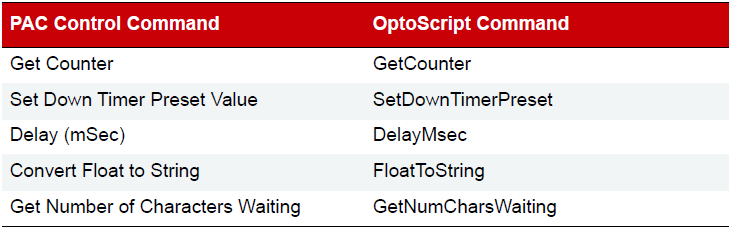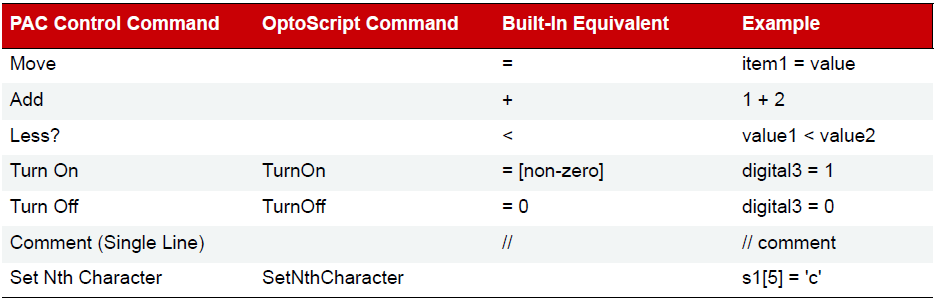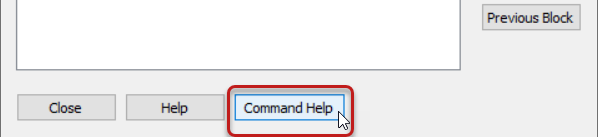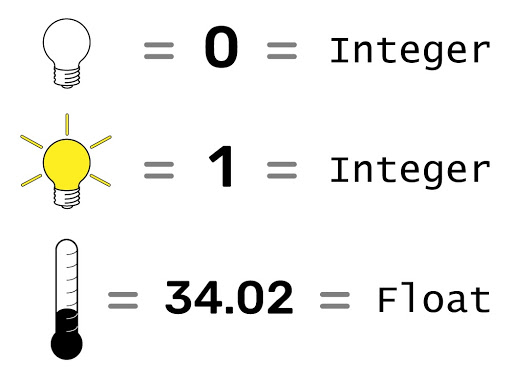## Functions and Commands

Functions in OptoScript are provided by commands almost identical to the standard commands in PAC Control. There are no additional functions for OptoScript code, and you can’t make your own functions.

Standard and OptoScript Commands
In many cases, you can easily recognize OptoScript commands because they’re almost the same as standard PAC Control commands.

• All spaces are removed from the OptoScript commands
• Words in the command are sometimes abbreviated or left out
• Commands are case sensitive

Here are some examples of the same commands in PAC Control and in OptoScript:Some commands are built into OptoScript functionality. Some of these have OptoScript commands and some don’t. You can use either the built-in functionality or the OptoScript command, if it exists.

Here are some examples:For more information, refer to the PAC Control User’s Guide, Appendix E: OptoScript Language Reference for a table of all PAC Control commands and their OptoScript equivalents. In addition, OptoScript equivalents for each command are shown in the PAC Control Command Reference manual and in the online Command Help.### Using I/O in OptoScript

One advantage of OptoScript is that any named I/O point can be used directly wherever a numeric variable can be used, rather than requiring a variable.

• Digital points behave like integer variables that have only two possible states: zero (off ) or non-zero (on).
• Analog points behave like float variables.For example, you can turn a digital output point off by simply assigning it a value of zero:

Outside_Light = 0;

You can turn a digital output point on by assigning it any value other than zero:

Outside_Light = 1;
Outside_Light = -1;
Outside_Light = 486;

You can use I/O points directly in mathematical expressions:

fLimit = Pressure_Input + 50;

Or use them directly in control structures, for example to turn off the light if the door is closed:

if (not Door) then     // Door is a digital input and Outside_Light is a digital output
Outside_Light = 0;
endif

You can set an output based on the value of an input or a variable:

LED01 = Switch_A;                // Switch_A is a digital input, and LED01 is digital output
Proportional_Valve = fPressure_Control;     // Proportional_Valve is an analog output
// and fPressure_Control is a float variable

You can use a point directly with a command:

fRange = GetAnalogMaxValue(Temp_Input) - GetAnalogMinValue(Temp_Input);
TurnOn(Fan_A);
IsOn(Fan_A);

#### Summary

Learn the basics of using functions and commands in OptoScript, as well as how to use I/O in OptoScript.